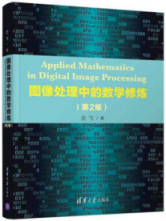# 医疗方面的图像处理

## 医疗图像处理与深度学习（二）

2019-06-18 15:55:17 weixin_37619439 阅读数 973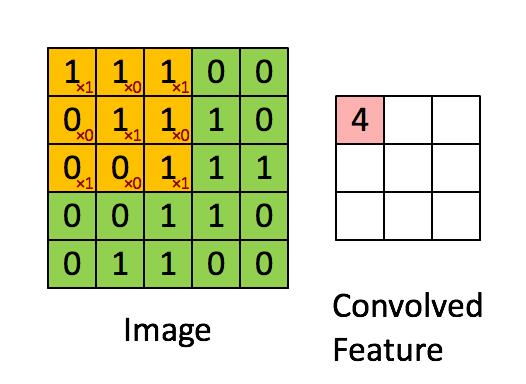Convolution with 3×3 Filter. Source: deeplearning.stanford.edu/wiki/index.php/Feature_extraction_using_convolution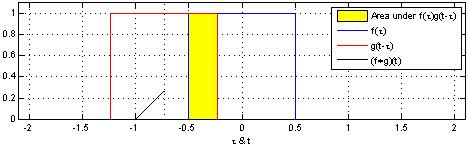Source: Wikipedia.

Jeremy Howard在他的MOOC中使用excel表解释了卷积，这是理解基本原理的好方法。 考虑2个矩阵f和g。 f和g的卷积输出是由2个矩阵的点积给出的第三个矩阵“Conv层1”。 2个矩阵的点积是标量，如下所示。 这里可以找到一个很好的数学函数源。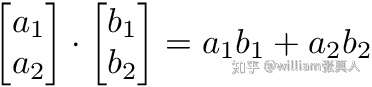Dot product of 2 matrices.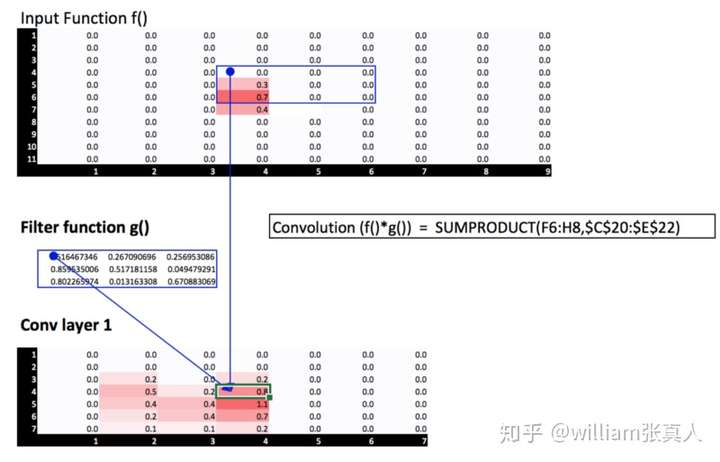Convolution of 2 matrices.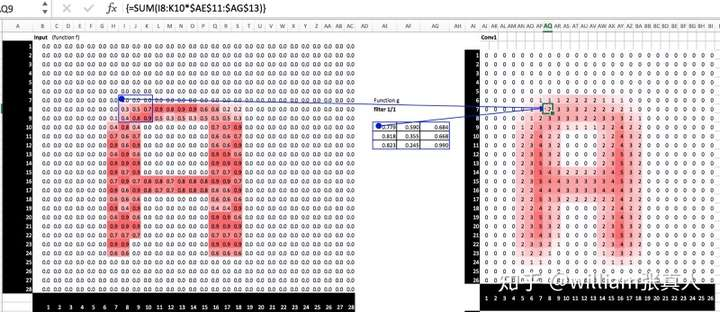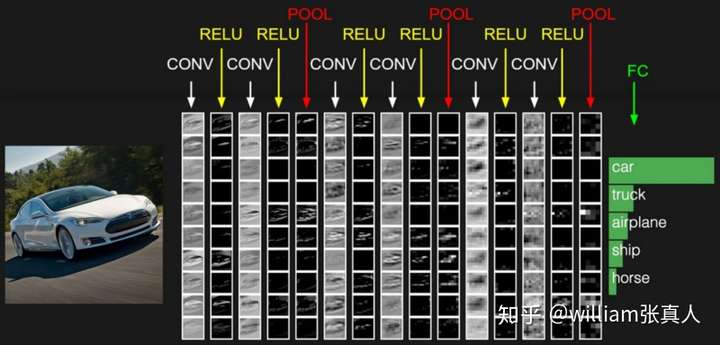Source: cs231n.github.io/convolutional-networks/

CNN的不同类型的实体是：输入，过滤器（或内核），卷积层，激活层，池化层和批量标准化层。 这些层以不同的排列组合，当然有些规则为我们提供了不同的深度学习架构。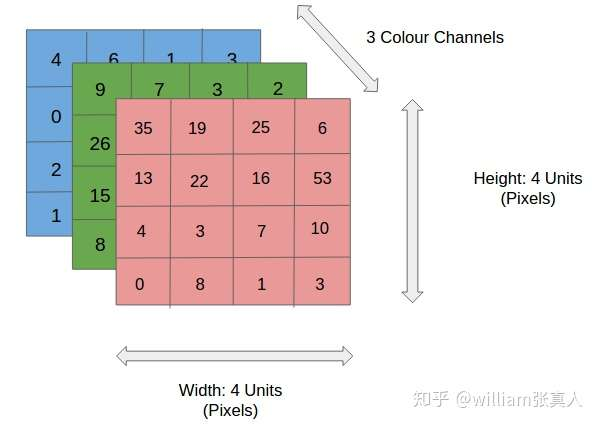Source: http://xrds.acm.org/blog/2016/06/convolutional-neural-networks-cnns-illustrated-explanation/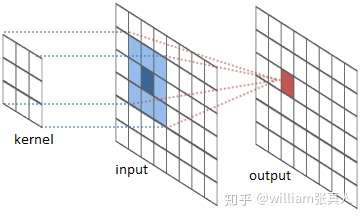source: intellabs.github.io/RiverTrail/tutorial/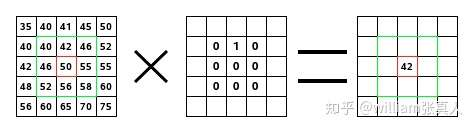Source: https://docs.gimp.org/en/plug-in-convmatrix.html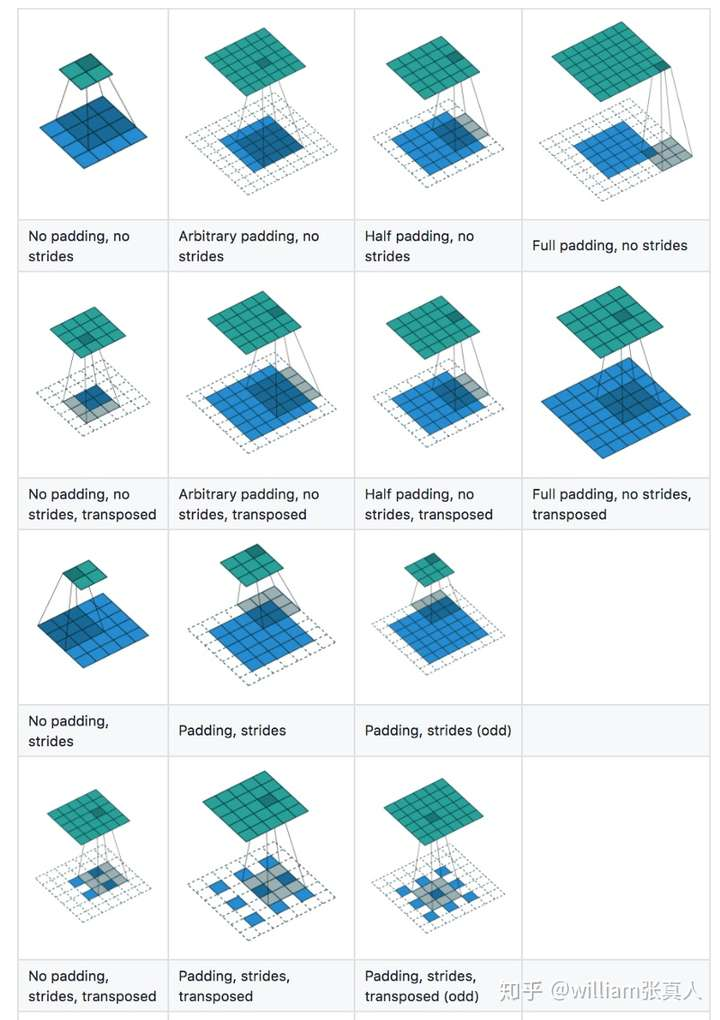Source: https://github.com/vdumoulin/conv_arithmetic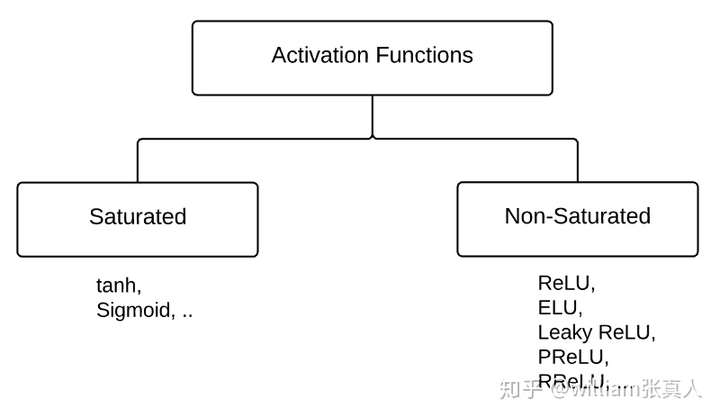Sigmoid：获取实值输入并将其压缩到[0,1]之间的范围

σ（x）= 1 /（1 + exp（-x））

tanh：获取实值输入并将其压缩到范围[-1,1]

tanh（x）=2σ（2x） - 1

RELU

ReLU全程叫整流线性单元。它是输入为x的最大函数（x，0），例如来自卷积图像的矩阵。然后，ReLU将矩阵x中的所有负值设置为零，并且所有其他值保持不变.ReLU在卷积之后计算，因此是非线性激活函数，如tanh或sigmoid。这是Geoff Hinton在他的nature报纸中首次讨论的。

ELUs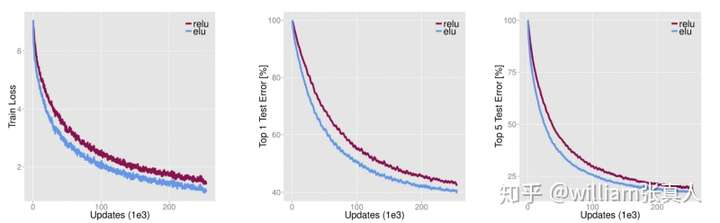Source: http://image-net.org/challenges/posters/JKU_EN_RGB_Schwarz_poster.pdf [15 layer CNN with stacks of (1×96×6, 3×512×3, 5×768×3, 3×1024×3, 2×4096×FC, 1×1000×FC) layers×units×receptive fields or fully-connected (FC). 2×2 max-pooling with a stride of 2 after each stack, spatia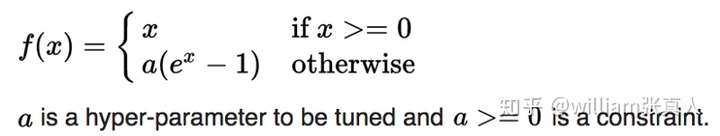Source: Wikipedia.

Leaky ReLUs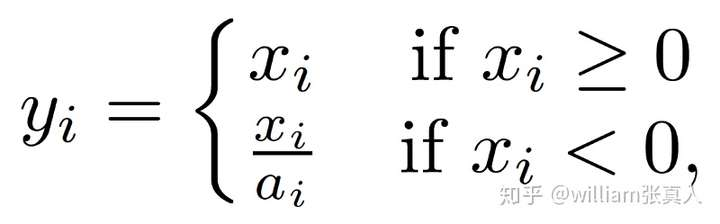Source: Empirical Evaluation of Rectified Activations in Convolution Network.

PReLU可以被视为Leaky ReLU的变种。 在PReLU中，负面部分的斜率是从数据中学习而不是预定义的。 作者声称，PReLU是超越ImageNet分类（Russakovsky等，2015）任务的人类表现的关键因素。 除了ai是通过反向传播在训练中学习，PReLU与ReLU相同。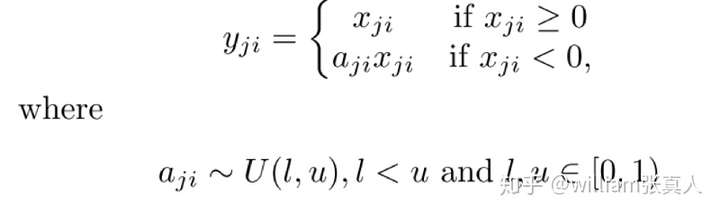ReLU，Leaky ReLU，PReLU和RReLU之间的比较如下所示。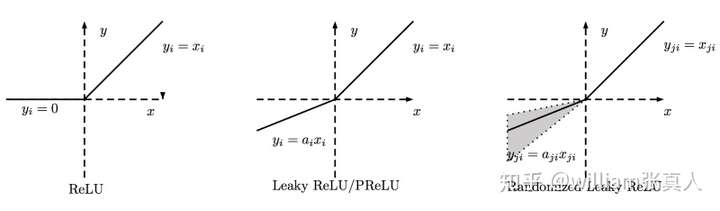Source :https://arxiv.org/pdf/1505.00853.pdf ReLU, Leaky ReLU, PReLU and RReLU. For PReLU, ai is learned and for Leaky ReLU ai is fixed. For RReLU, aji is a random variable keeps sampling in a given range, and remains fixed in testing.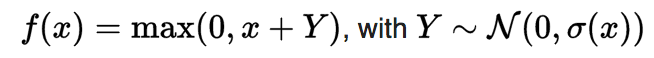Source: Wikipedia.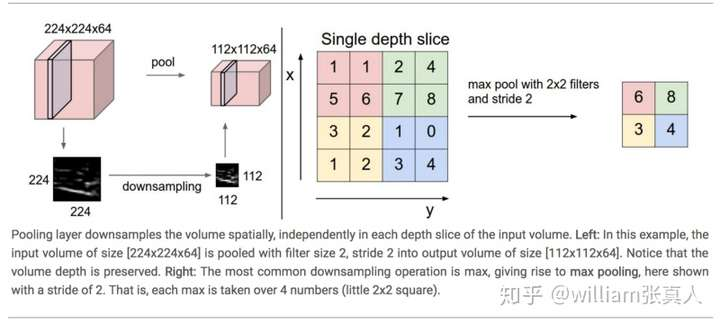Source: http://cs231n.github.io/convolutional-networks/#pool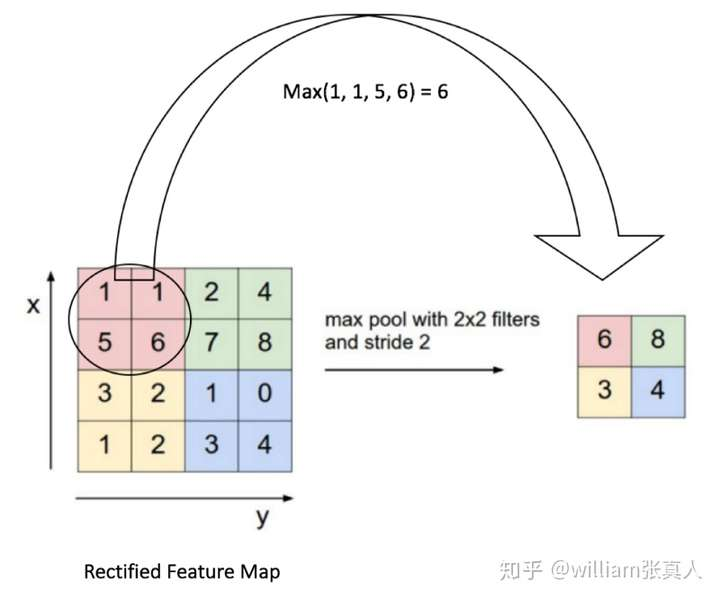Source: https://ujjwalkarn.me/2016/08/11/intuitive-explanation-convnets/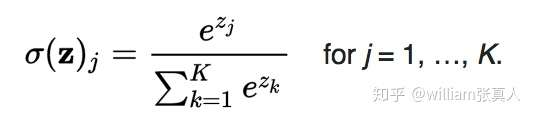Source: Wikipedia

Softmax激活通常用于最终的完全连接层，以获得概率，它的值为0到1之间。

## 常见医疗扫描图像处理步骤

2017-06-09 15:02:13 u013635029 阅读数 11934

## 一 下载必要包

skimage需要更新到最新0.13版本，否则会报错，ImportError: cannot import name label。

``sudo pip install scikit-image  -U -i  https://pypi.tuna.tsinghua.edu.cn/simple``

## 二 数据格式

2.1 dicom``dicom.read_file('/data/lung_competition/stage1/7050f8141e92fa42fd9c471a8b2f50ce/498d16aa2222d76cae1da144ddc59a13.dcm')``

``````slices = [dicom.read_file(os.path.join(folder_name,filename)) for filename in os.listdir(folder_name)]
slices = np.stack([s.pixel_array for s in slices])``````

2.2 mhd格式

mhd格式是另外一种数据格式，来源于(LUNA2016)[https://luna16.grand-challenge.org/data/]。每个病人一个mhd文件和一个同名的raw文件。如下:``````import SimpleITK as sitk
img_array = sitk.GetArrayFromImage(itk_img) # indexes are z,y,x (notice the ordering)
num_z, height, width = img_array.shape        #heightXwidth constitute the transverse plane
origin = np.array(itk_img.GetOrigin())      # x,y,z  Origin in world coordinates (mm)
spacing = np.array(itk_img.GetSpacing())    # spacing of voxels in world coor. (mm)``````

2.3 查看CT扫描文件软件## 三 dicom格式数据处理过程

3.1 处理思路``Hounsfield Unit = pixel_value * rescale_slope + rescale_intercept``

LUT全称look up table，实际上就是一张像素灰度值的映射表，它将实际采样到的像素灰度值经过一定的变换如阈值、反转、二值化、对比度调整、线性变换等，变成了另外一 个与之对应的灰度值，这样可以起到突出图像的有用信息，增强图像的光对比度的作用。

slices[slices == -2000] = 0

3.2 先载入必要的包

``````# -*- coding:utf-8 -*-
'''
this script is used for basic process of lung 2017 in Data Science Bowl
'''

import glob
import os
import pandas as pd
import SimpleITK as sitk

import numpy as np # linear algebra
import pandas as pd # data processing, CSV file I/O (e.g. pd.read_csv)
import skimage, os
from skimage.morphology import ball, disk, dilation, binary_erosion, remove_small_objects, erosion, closing, reconstruction, binary_closing
from skimage.measure import label,regionprops, perimeter
from skimage.morphology import binary_dilation, binary_opening
from skimage.filters import roberts, sobel
from skimage import measure, feature
from skimage.segmentation import clear_border
from skimage import data
from scipy import ndimage as ndi
import matplotlib
#matplotlib.use('Agg')
import matplotlib.pyplot as plt
from mpl_toolkits.mplot3d.art3d import Poly3DCollection
import dicom
import scipy.misc
import numpy as np``````

DICOM是医学图像中标准文件，这些文件包含了诸多的

``````# Load the scans in given folder path
slices = [dicom.read_file(path + '/' + s) for s in os.listdir(path)]
slices.sort(key = lambda x: int(x.ImagePositionPatient))
try:
slice_thickness = np.abs(slices.ImagePositionPatient - slices.ImagePositionPatient)
except:
slice_thickness = np.abs(slices.SliceLocation - slices.SliceLocation)

for s in slices:
s.SliceThickness = slice_thickness

return slices``````

3.3 像素转换为HU单元

``````def get_pixels_hu(slices):
image = np.stack([s.pixel_array for s in slices])
# Convert to int16 (from sometimes int16),
# should be possible as values should always be low enough (<32k)
image = image.astype(np.int16)
# Set outside-of-scan pixels to 0
# The intercept is usually -1024, so air is approximately 0
image[image == -2000] = 0

``````
``````# Convert to Hounsfield units (HU)
for slice_number in range(len(slices)):

intercept = slices[slice_number].RescaleIntercept
slope = slices[slice_number].RescaleSlope

if slope != 1:
image[slice_number] = slope * image[slice_number].astype(np.float64)
image[slice_number] = image[slice_number].astype(np.int16)

image[slice_number] += np.int16(intercept)

return np.array(image, dtype=np.int16)``````

``````first_patient = load_scan(INPUT_FOLDER + patients)
first_patient_pixels = get_pixels_hu(first_patient)
plt.hist(first_patient_pixels.flatten(), bins=80, color='c')
plt.xlabel("Hounsfield Units (HU)")
plt.ylabel("Frequency")
plt.show()``````3.4重新采样

``````def resample(image, scan, new_spacing=[1,1,1]):
# Determine current pixel spacing
spacing = map(float, ([scan.SliceThickness] + scan.PixelSpacing))
spacing = np.array(list(spacing))
resize_factor = spacing / new_spacing
new_real_shape = image.shape * resize_factor
new_shape = np.round(new_real_shape)
real_resize_factor = new_shape / image.shape
new_spacing = spacing / real_resize_factor

image = scipy.ndimage.interpolation.zoom(image, real_resize_factor, mode='nearest')

return image, new_spacing``````

``pix_resampled, spacing = resample(first_patient_pixels, first_patient, [1,1,1])``

``````def plot_3d(image, threshold=-300):

# Position the scan upright,
# so the head of the patient would be at the top facing the camera
p = image.transpose(2,1,0)

verts, faces = measure.marching_cubes(p, threshold)
fig = plt.figure(figsize=(10, 10))
# Fancy indexing: `verts[faces]` to generate a collection of triangles
mesh = Poly3DCollection(verts[faces], alpha=0.1)
face_color = [0.5, 0.5, 1]
mesh.set_facecolor(face_color)
ax.set_xlim(0, p.shape)
ax.set_ylim(0, p.shape)
ax.set_zlim(0, p.shape)
plt.show()``````

``plot_3d(pix_resampled, 400)``3.5 输出一个病人scans中所有切面slices

``````def plot_ct_scan(scan):
'''
plot a few more images of the slices
:param scan:
:return:
'''
f, plots = plt.subplots(int(scan.shape / 20) + 1, 4, figsize=(50, 50))
for i in range(0, scan.shape, 5):
plots[int(i / 20), int((i % 20) / 5)].axis('off')
plots[int(i / 20), int((i % 20) / 5)].imshow(scan[i], cmap=plt.cm.bone)``````3.6 定义分割出CT切面里面肺部组织的函数

``````def get_segmented_lungs(im, plot=False):

'''
This funtion segments the lungs from the given 2D slice.
'''
if plot == True:
f, plots = plt.subplots(8, 1, figsize=(5, 40))
'''
Step 1: Convert into a binary image.
'''
binary = im < 604
if plot == True:
plots.axis('off')
plots.set_title('binary image')
plots.imshow(binary, cmap=plt.cm.bone)

'''
Step 2: Remove the blobs connected to the border of the image.
'''
cleared = clear_border(binary)
if plot == True:
plots.axis('off')
plots.set_title('after clear border')
plots.imshow(cleared, cmap=plt.cm.bone)

'''
Step 3: Label the image.
'''
label_image = label(cleared)
if plot == True:
plots.axis('off')
plots.set_title('found all connective graph')
plots.imshow(label_image, cmap=plt.cm.bone)
'''
Step 4: Keep the labels with 2 largest areas.
'''
areas = [r.area for r in regionprops(label_image)]
areas.sort()
if len(areas) > 2:
for region in regionprops(label_image):
if region.area < areas[-2]:
for coordinates in region.coords:
label_image[coordinates, coordinates] = 0
binary = label_image > 0
if plot == True:
plots.axis('off')
plots.set_title(' Keep the labels with 2 largest areas')
plots.imshow(binary, cmap=plt.cm.bone)
'''
Step 5: Erosion operation with a disk of radius 2. This operation is
seperate the lung nodules attached to the blood vessels.
'''
selem = disk(2)
binary = binary_erosion(binary, selem)
if plot == True:
plots.axis('off')
plots.set_title('seperate the lung nodules attached to the blood vessels')
plots.imshow(binary, cmap=plt.cm.bone)
'''
Step 6: Closure operation with a disk of radius 10. This operation is
to keep nodules attached to the lung wall.
'''
selem = disk(10)
binary = binary_closing(binary, selem)
if plot == True:
plots.axis('off')
plots.set_title('keep nodules attached to the lung wall')
plots.imshow(binary, cmap=plt.cm.bone)
'''
Step 7: Fill in the small holes inside the binary mask of lungs.
'''
edges = roberts(binary)
binary = ndi.binary_fill_holes(edges)
if plot == True:
plots.axis('off')
plots.set_title('Fill in the small holes inside the binary mask of lungs')
plots.imshow(binary, cmap=plt.cm.bone)
'''
Step 8: Superimpose the binary mask on the input image.
'''
get_high_vals = binary == 0
im[get_high_vals] = 0
if plot == True:
plots.axis('off')
plots.set_title('Superimpose the binary mask on the input image')
plots.imshow(im, cmap=plt.cm.bone)

return im``````3.7 肺部图像分割

• 阈值图像（-320HU是个极佳的阈值，但是此方法中不是必要）
• 处理相连的组件，以决定当前患者的空气的标签，以1填充这些二值图像
• 可选：当前扫描的每个轴上的切片，选定最大固态连接的组织（当前患者的肉体和空气），并且其他的为0。以掩码的方式填充肺部结构。
• 只保留最大的气袋（人类躯体内到处都有气袋）
``````def largest_label_volume(im, bg=-1):
vals, counts = np.unique(im, return_counts=True)
counts = counts[vals != bg]
vals = vals[vals != bg]
if len(counts) > 0:
return vals[np.argmax(counts)]
else:
return None

# not actually binary, but 1 and 2.
# 0 is treated as background, which we do not want
binary_image = np.array(image > -320, dtype=np.int8)+1
labels = measure.label(binary_image)

# Pick the pixel in the very corner to determine which label is air.
#   Improvement: Pick multiple background labels from around the patient
#   More resistant to "trays" on which the patient lays cutting the air
#   around the person in half
background_label = labels[0,0,0]

#Fill the air around the person
binary_image[background_label == labels] = 2

# Method of filling the lung structures (that is superior to something like
# morphological closing)
if fill_lung_structures:
# For every slice we determine the largest solid structure
for i, axial_slice in enumerate(binary_image):
axial_slice = axial_slice - 1
labeling = measure.label(axial_slice)
l_max = largest_label_volume(labeling, bg=0)

if l_max is not None: #This slice contains some lung
binary_image[i][labeling != l_max] = 1

binary_image -= 1 #Make the image actual binary
binary_image = 1-binary_image # Invert it, lungs are now 1

# Remove other air pockets insided body
labels = measure.label(binary_image, background=0)
l_max = largest_label_volume(labels, bg=0)
if l_max is not None: # There are air pockets
binary_image[labels != l_max] = 0

return binary_image``````

``````segmented_lungs = segment_lung_mask(pix_resampled, False)
plot_3d(segmented_lungs, 0)````````plot_3d(segmented_lungs_fill, 0)``3.8 数据预处理

``````#归一化
MIN_BOUND = -1000.0
MAX_BOUND = 400.0

def normalize(image):
image = (image - MIN_BOUND) / (MAX_BOUND - MIN_BOUND)
image[image>1] = 1.
image[image<0] = 0.
return image``````

0值中心化

``````PIXEL_MEAN = 0.25

def zero_center(image):
image = image - PIXEL_MEAN
return image
``````

3.9 存储每个病人scan的所有slice肺部特征

`````` lung_head_dir = '/data/lung_competition/stage1/'
#patient_labels_file = '/data/lung_competition/stage1_labels.csv'
selem = ball(2)
#print(patient_scans_list)
for scan in patient_scans_list:
if os.path.exists(save_npy_path):
os.mkdir(save_npy_path)``````

``````segmented_ct_scan = segment_lung_from_ct_scan(ct_scan)
save_npy = save_npy_path+'.npy'
np.save(save_npy,segmented_ct_scan)
print('file %s saved ..'%save_npy)
plot_ct_scan(segmented_ct_scan)``````

## 四 mhd格式数据处理过程

4.1 处理思路

mhd的数据只是格式与dicom不一样，其实质包含的都是病人扫描。处理MHD需要借助SimpleIKT这个包，处理思路详情可以参考Data Science Bowl2017的toturail Data Science Bowl 2017。需要注意的是MHD格式的数据没有HU值，它的值域范围与dicom很不同。

4.2 载入必要的包

``````import SimpleITK as sitk
import numpy as np
import csv
from glob import glob
import pandas as pd
file_list=glob(luna_subset_path+"*.mhd")
#####################
#
# Helper function to get rows in data frame associated
# with each file
def get_filename(case):
global file_list
for f in file_list:
if case in f:
return(f)
#
# The locations of the nodes
df_node["file"] = df_node["seriesuid"].apply(get_filename)
df_node = df_node.dropna()
#####
#
# Looping over the image files
#
fcount = 0
for img_file in file_list:
print "Getting mask for image file %s" % img_file.replace(luna_subset_path,"")
mini_df = df_node[df_node["file"]==img_file] #get all nodules associate with file
if len(mini_df)>0:       # some files may not have a nodule--skipping those
biggest_node = np.argsort(mini_df["diameter_mm"].values)[-1]   # just using the biggest node
node_x = mini_df["coordX"].values[biggest_node]
node_y = mini_df["coordY"].values[biggest_node]
node_z = mini_df["coordZ"].values[biggest_node]
diam = mini_df["diameter_mm"].values[biggest_node]``````

4.3 LUNA16的MHD格式数据的值

4.4 坐标体系变换

MHD值得坐标体系是体素，以mm为单位。结节的位置是CT scanner坐标轴里面相对原点的mm值，需要将其转换到真实坐标轴位置，可以使用SimpleITK包中的 GetOrigin() `GetSpacing()`。图像数据是以512x512数组的形式给出的。``````k_img = sitk.ReadImage(img_file)
img_array = sitk.GetArrayFromImage(itk_img) # indexes are z,y,x (notice the ordering)
center = np.array([node_x,node_y,node_z])   # nodule center
origin = np.array(itk_img.GetOrigin())      # x,y,z  Origin in world coordinates (mm)
spacing = np.array(itk_img.GetSpacing())    # spacing of voxels in world coor. (mm)
v_center =np.rint((center-origin)/spacing)  # nodule center in voxel space (still x,y,z ordering)
``````

``````i=0
for i_z in range(int(v_center)-1,int(v_center)+2):
imgs[i] = matrix2int16(img_array[i_z])
i+=1
np.save(output_path+"images_%d.npy" % (fcount) ,imgs)

4.5 查看结节

``````import matplotlib.pyplot as plt
for i in range(len(imgs)):
print "image %d" % i
fig,ax = plt.subplots(2,2,figsize=[8,8])
ax[0,0].imshow(imgs[i],cmap='gray')
plt.show()
raw_input("hit enter to cont : ")``````4.6 包含结节位置信息的mhd格式数据特征

## 【算法】图像处理在医学领域的应用

2019-10-02 16:03:57 qq_41985559 阅读数 789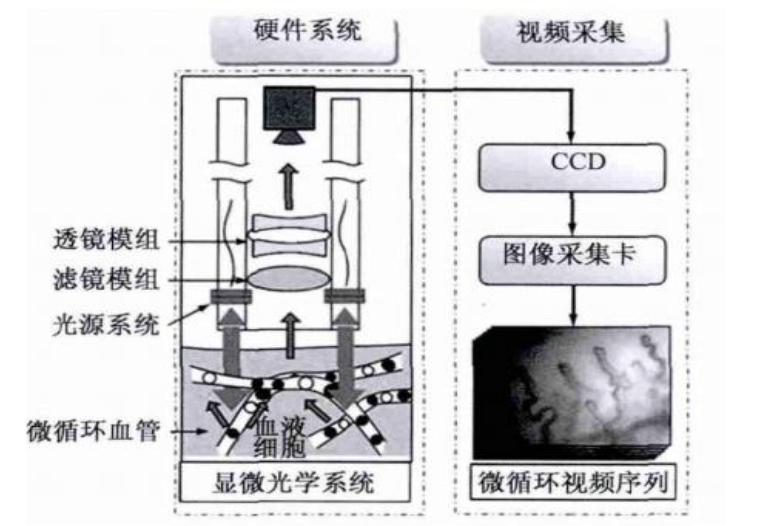-滤波反投影算法：

1. ``````计算每一个投影的一维傅里叶变换；
``````
2. ``````用滤波函数 乘以每一个傅里叶变换；
``````
3. ``````得到每一个滤波后的变换的一维反傅里叶变换；
``````
4. ``````对步骤3得到的所以一维反变换积分（求和）
``````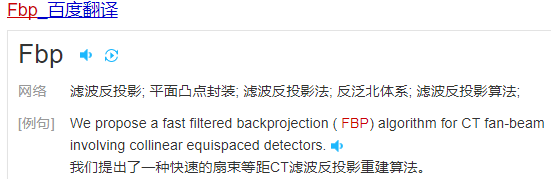X光特别适合用于骨骼外伤，如果怀疑伤到了骨头，优选X光，检查结果快速易得；也适用于胸部的粗略检查，X光胸片可粗略检查心脏、主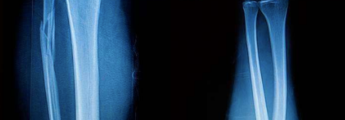CT是用X射线束对人体某部位一定厚度地扫描，根据人体不同组织对X线的吸收与透过率的不同，应用灵敏度极高的仪器对人体进行测量，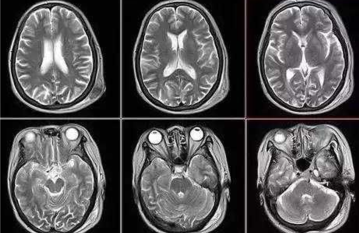B超的原理是用超声波穿透人体，当声波遇到人体组织时会产生反射波，通过计算反射波成像。就像挑西瓜一样，边敲边看显示病灶情况。B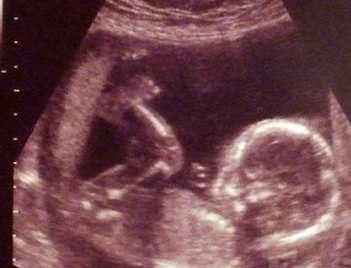## 图像处理在医学方面的应用

2018-11-24 17:06:23 weixin_43789163 阅读数 3085

## 浅谈图像处理在医学方面的应用

1、背景：

2、医学图像处理技术：

（1） 图像分割：

（2） 图像配准和图像融合：

（3） 伪彩色处理技术：

3、总结：

4、参考文献：
［1］王新成.高级图像处理技术［M］.北京：中国科学技术出版社，2001.
［2］丁莹.图像配准技术在医学图像处理中的应用研究［M］.长春理工大学，2006.12.
［3］田捷.医学影像处理与分析[M], 电子工业出版社, 2003.
［4］田娅, 饶妮妮, 蒲立新.国内医学图像处理技术的最新动态[J].电子科技大学学报, 2002(5): 485- 489.

## 如何学好图像处理——从小白到大神？

2016-02-26 17:48:13 baimafujinji 阅读数 70342

1950年，美国的麻省理工学院制造出了第一台配有图形显示器的电子计算机——旋风I号（Whirlwind I）。旋风I号的显示器使用一个类似于示波器的阴极射线管（Cathode Ray Tube，CRT）来显示一些简单的图形。1958年美国Calcomp公司研制出了滚筒式绘图仪，GerBer公司把数控机床发展成为平板式绘图仪。在这一时期，电子计算机都主要应用于科学计算，而为这些计算机配置的图形设备也仅仅是作为一种简单的输出设备。

1964年，美国加利福尼亚的喷气推进实验室用计算机对“旅行者七号”太空船发回的大批月球照片进行处理，以校正航天器上摄影机中各种类型的图像畸变，收到了明显的效果。在后来的宇航空间技术中，数字图像处理技术都发挥了巨大的作用。

• 1）图像获取和输出
• 2）图像编码和压缩
• 3）图像增强与复原
• 4）图像的频域变换
• 5）图像的信息安全
• 6）图像的区域分割
• 7）图像目标的识别
• 8）图像的几何变换

• 1）一些专业图像处理软件：Photoshop、CorelDRAW……
• 2）一些手机APP应用：美图秀秀、玩图……
• 3）一些医学图像处理应用：MRI、彩超图像处理……
• 4）一些制造业上的应用：元器件检测、瑕疵检测……
• 5）一些摄像头、相机上的应用：夜间照片的质量改善……
• 6）一些电影工业上是应用：换背景、电影特技……

1）如果你是我上述那些应用领域的从业者，你当然需要掌握图像方面的理论和技术；2）相关专业的研究人员、大专院校的博士生、研究生。

1）对于初级入门者

1）这是一本专门为Electronic Engineering专业学生所写的书。它需要有信号与系统、数字信号处理这两门课作为基础。如果你没有这两门课的基础，你读这本书要么是看热闹，要么就是看不懂。2）冈萨雷斯教授的《数字图像处理》第一版是在1977年出版的，到现在已经快40年了；现在国内广泛使用的第二版是2002年出版的（第三版是2007年但是其实二者差异并不大），到现在也有20年左右的时间了。事实上，冈萨雷斯教授退休也有快30年了。所以这本书的内容已经偏于陈旧。数字图像处理这个领域的发展绝对是日新月异，突飞猛进的。特别在最近二三十年里，很多新思路，新方法不断涌现。如果你看了我前面推荐的Rich教授的公开课（这也是当前美国大学正在教学的内容），你一下子就会发现，原来我们的教育还停留在改革开放之前外国的水平上。这其实特别可怕。所以我觉得冈萨雷斯教授的《数字图像处理》作为学习过程中的一个补充还是不错的，但是如果把它作为主参考，那真的就是：国外都洋枪洋炮了，我们还在大刀长矛。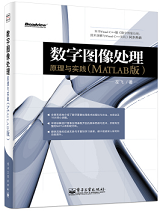2）对于中级水平者

http://blog.csdn.net/baimafujinji/article/details/32332079

3）对于高级进阶者

（全文完）